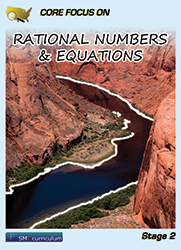#### Rational Numbers & Equations

Students apply and extend previous understandings of operations with fractions to add, subtract, multiply, and divide rational numbers.  They use properties of operations to generate equivalent expressions. Students learn to solve real-life and mathematical problems using numerical and algebraic expressions and equations.

### Textbook & Stage I Series Pricing

1 FREE Teacher Edition(TE) and Teacher Resource Binder(TR) per 40 student texts ordered!
2Item CodePublisher NumberBook TitleTypeCopyrightPrice
97819388016791938801679Core Focus on Math:Stage 2 Mathematics SetTX2014\$64.95
97819388017301938801733Core Focus on Rational Numbers/EquationsTX2014\$27.95
97819388016001938801601Core Focus on Rational Numbers/Equations TETE2014\$32.95
97819350335851935033581Core Focus on Rational Numbers/Equations TRTR2013\$99.95